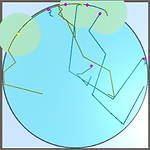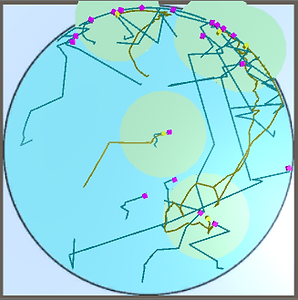top of page

## 1. Cooperative Multi-Robot Observation of Multiple Moving Targets

###### Scenairo

m = 3 holonomic point robots with 360-degree field of view sensors of range do3 = 30 m must observe n = 6 holonomic targets moving randomly within a circular environment of radius R = 100 m. The speed of each target is fixed and randomly chosen to be between 0 m/s and 1.5 m/s. The maximum speed of each robot is 2 m/s. Assume that the sensing of each robot is perfect and that each robot may communicate with other robots throughout the environment. The goal of the robots is to maximize the average number of targets that are being observed by at least one robot at each time step dt = 1 throughout the mission of length T = 120 s.

Implementing the algorithm from

###### Simulation Environment

Unity Engine is used as the tool for simulation the behavior of this cooperative multi-robot observation of moving targets task. Figure 1 (below) basically shows the simulation environment. The left-side window, which is the Scene View, shows that models and environment are created in a three-dimensional virtual world. So this could provide possibility for further 3D usage if necessary. The black circle is the simulation field with a radius of 100 m.

The right-side window is the actual Game View, which displays the simulation process. To be specific, the six purple dots are the six targets. Their initial positions will have no effect to the results because their initial positions will be randomly placed once the program starts. The three yellow dots, which locate inside three green circles, are the robots. Each green circle is the sensing range for each corresponding robot. The sensing range, in this case, is 30 m. The start positions of the robots are fixed in the experiment. Assuming the center of the black circle is (0,0), the positions of the robots are (0, 50), (-43.3, -25) and (43.3, 25), respectively.

The simulation environment is finished by the author by editing with Unity and scripting with C#.###### Experiment

Figure 1 Simulation environment (i.e. Unity3D Engine editor interface).###### Baseline Case

Figure 2 Baseline cases: (a), (b), (c), (d) and (e) from left to right.

Table 1 Average covered target numbers during 120s of the baseline cases.###### Standard case (3 robots, 6 targets, m/n: 1/2):Figure 3 Simulation results of 3 robots and 6 targets, with targets moving random/linearly.

Table 2 Average covered target numbers during 120s of the 3 robots and 6 targets case.Note: f, g, h and i indicate the case that locate in left-top, right-top, left-bottom and right-bottom figures in Figure 3, respectively.

###### Another case (5 robots, 20 targets, m/n: 1/4):

Figure 4 Simulation results of 5 robots and 20 targets, with targets moving random/linearly.

Table 4 Average covered target numbers during 120s of the 5 robots and 20 targets case.

Note: j, k, l and m indicate the case that locate in left-top, right-top, left-bottom and right-bottom figures in Figure 4, respectively.###### Increase the radius of the environment: 100m, 200m, 500 mFigure 5 Simulation results of 3 robots and 6 targets, with targets moving random/linearly, when the radius of the environment is 100 m (left), 200m (middle) and 500 m (right), respectively.

Table 5 Average covered target numbers during 120s of the 3 robots and 6 targets cases when increasing the radius of the environment of 100 m, 200 m and 500 m.The results from both Table 3 and Figure 4 show that the radius of the environment is a dominant factor in the experiment when keeping the numbers of the targets and the robots invariant. When doubled the radius of the environment from 100 m to 200 m, the average covered target numbers during 120s dropped greatly from 3.762 to 0.926. Moreover, with increasing the radius to 500 m, the average covered target numbers during 120s is even 0, which indicates that in such a large environment, the robots are almost impossible to find the targets with current algorithm and amount of robots and targets.

bottom of page